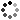# 7th Grade Math Higher Order Thinking Skills Activity - What's the Question

On Sale
\$4.50
\$4.50
Preview

IEncourage your 7th grade math students to use their higher-order thinkig skills in this activity. Students will critically think as they develop the question the the given answer. This includes various 7th grade math topics such as: integers, equations, expressions, unit rates, proportional relationships and more.

This product consists of 14 different topics. There is one topic per page. Each topic consists of 5 different “questions.” The questions are actually the answers given to five different problems, and the students have to create the questions. This will help your students think deeper about the mathematics.

The 14 topics include:
• Subtracting Integers
• Multiplying and Dividing Integers
• Simplifying Expressions: Simplifying expressions with rational numbers and the distributive property.
• Equations: Solving equations with rational numbers and the distributive property.
• Unit Rates: Unit rates with rational numbers.
• Proportional Relationships: Proportional relationships in tables, graphs and equations.
• Constant of Proportionality: Identifying constants of proportionality in tables, graphs, and equations.
• Triangles: Angles and side lengths that form a triangle.
• Circles: Circumference and area of circles
• Surface Area and Volume: Surface Area and volume of right rectangular prisms and pyramids.
• Slicing: Identifying a two-dimensional figure from slicing a three-dimensional shape.
• Statistics: Comparing means and medians, identifying a random sample, and bias questions.
• Probability: Probability between 0 and 1 and uniform vs. non-uniform probability models.
An answer sheet is NOT included. Due to the nature of the activity, the answers will widely vary. I have included sample answers for various questions to give the teacher an idea of what to accept. A suggestion for the teacher: do not be so strict on students writing the right “answer” for this activity. Rather this is to challenge students to think beyond basic algorithms. Focus on the thinking of the students and you can learn a great deal on what your students really understand.

These would be great to use for assessments, homework, math centers, group work, or individual work.

Please feel free to contact me with any questions!

Michelle,
Make Sense of Math